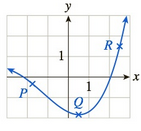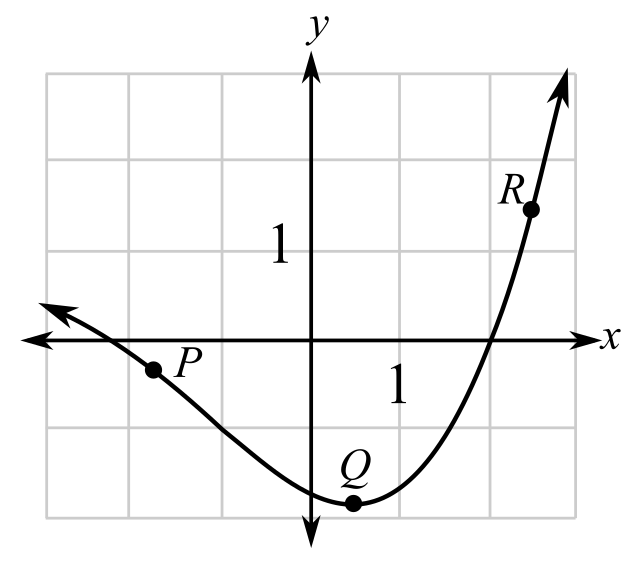Chapter 10.5, Problem 23EFinite Mathematics and Applied Cal...

7th Edition
Stefan Waner + 1 other
ISBN: 9781337274203

Solutions

Chapter
SectionFinite Mathematics and Applied Cal...

7th Edition
Stefan Waner + 1 other
ISBN: 9781337274203
Textbook Problem

In each of Exercises 23–26, three slopes are given. For each slope, determine at which of the labeled points on the graph the tangent line has that slope.a. 0 b. 4 c. − 1(a)

To determine

The labelled points on the graph that has a value of the slope of a tangent as 0.Explanation

Given information:

The provided graph:

When a function is increasing or rising the slope of the function is positive and greatest where the tangent is more slanted whereas when the function is decreasing or...

(b)

To determine

The labelled points on the graph that has a value of the slope of a tangent as 4.(c)

To determine

The labelled points on the graph that has a value of the slope of the tangent is 1.Still sussing out bartleby?

Check out a sample textbook solution.

See a sample solution

The Solution to Your Study Problems

Bartleby provides explanations to thousands of textbook problems written by our experts, many with advanced degrees!

Get Started

Find the mean, median, and mode for the following scores; 8, 7, 5, 7, 0, 10, 2, 4, 11, 7, 8, 7

Essentials of Statistics for The Behavioral Sciences (MindTap Course List)

1340 Calculate y. y=xcosx

Calculus (MindTap Course List)

Find the maximum profit P (in dollars) given that 6(P 2500) 4(P + 2400)

Applied Calculus for the Managerial, Life, and Social Sciences: A Brief Approach

Evaluate the integral. 0(5ex+3sinx)dx

Single Variable Calculus: Early Transcendentals

For , f(x) = 0 2 3 f(3) does not exist

Study Guide for Stewart's Multivariable Calculus, 8th

limx0+(1+x)1/x= a) e b) e c) 1e d) 1e

Study Guide for Stewart's Single Variable Calculus: Early Transcendentals, 8th